Do you like the article?
Share it with others -
Use new possibilities of MetaTrader 5

# Neural networks made easy (Part 12): Dropout

23 April 2021, 11:23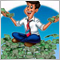0
6 885

### Introduction

Since the beginning of this series of articles, we have already made a big progress in studying various neural network models. But the learning process was always performed without our participation. At the same time, there is always a desire to somehow help the neural network to improve training results, which can also be referred to as the convergence of the neural network. In this article we will consider one of such methods entitled Dropout.

### 1. Dropout: A Method for Increasing Neural Network Convergence

When training a neural network, a large number of features are fed into each neuron, and it is difficult to evaluate the impact of each separate feature. As a result, the errors of some neurons are smoothed out by the correct values of other neurons, while these errors are accumulated at the neural network output. This causes the training to stop at a certain local minimum with a rather large error. This effect is referred to as the co-adaptation of feature detectors, in which the influence of each feature adjusts to the environment. It would be better to have the opposite effect, when the environment is decomposed into separate features and it is possible to evaluate the influence of each feature separately.

In 2012, a group of scientists from the university of Toronto proposed to randomly exclude some of the neurons from the learning process as a solution to the complex co-adaptation problem . A decrease in the number of features in training increases the importance of each feature, and a constant change in the quantitative and qualitative composition of features reduces the risk of their co-adaptation. This method is called Dropout. Sometimes the application of this method is compared to decision trees: by dropping out some of the neurons, we obtain a new neural network with its own weights at each training iteration. According to the combinatorics rules, such networks have quite a high variability.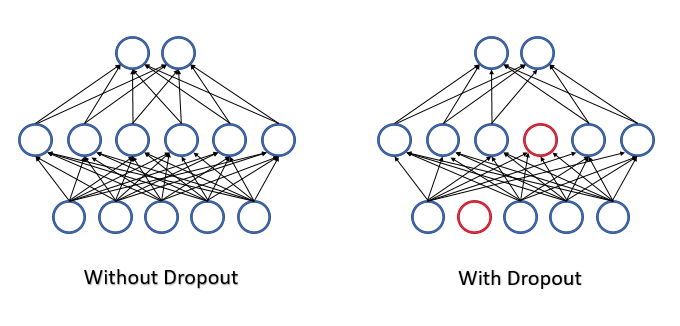All features and neurons are assessed during neural network operation and thus we obtain the most accurate and independent assessment of the current state of the analyzed environment.

The authors mention in their article (12) the possibility of using the method in order to increase the quality of pre-trained models.

From the point of view of mathematics, we can describe this process as dropping out each individual neuron out of the process with a certain given probability p. In other words, the neuron will participate in the neural network learning process with the probability of q=1-p.

The list of neurons which will be excluded is determined by a pseudo-random number generator with a normal distribution. This approach allows achieving the maximum possible uniform exclusion of neurons. We will generate in practice a vector with a size equal to the input sequence. "1" in the vector will be used for the feature to be used in training, and "0" will be used for the elements to be excluded.

However, the exclusion of the analyzed features undoubtedly leads to a decrease in the amount at the input of the neuron activation function. To compensate for this effect, we will multiply the value of each feature by the 1/q coefficient. This coefficient will increase the values because probability q will always be in the range between 0 and 1.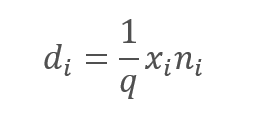where:

d — elements of the Dropout result vector,

q — the probability of using the neuron in the training process,

x — elements of the masking vector,

n — elements of the input sequence.

In the feed-backward pass during the learning process, the error gradient is multiplied by the derivative of the above function. As you can see, in the case of Dropout, the feed-backward pass will be similar to the feed-forward pass using the masking vector from the feed-forward pass.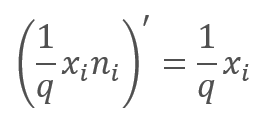During the operation of the neural network, the masking vector is filled with "1", which allows values to be passed smoothly in both directions.

In practice, the coefficient 1/q is constant throughout the entire training, so we can easily calculate this coefficient once and write it instead of "1" into the masking tensor. Thus, we can exclude the operations of recalculating the coefficient and multiplying it by "1" of the mask in each training iteration.

### 2. Implementation

Now that we have considered the theoretical aspects, let us move on to considering the variants for implementing this method in our library. The first thing we come across is the implementation of two different algorithms. One of them is needed for the training process and the second one will be used for production. Accordingly, we need to explicitly indicate to the neuron according to which algorithm it should work in each individual case. For this purpose, we will introduce the bTrain flag at the level of the base neuron. The flag value will be set to true for training and to false for testing.

class CNeuronBaseOCL    :  public CObject
{
protected:
bool               bTrain;             ///< Training Mode Flag



The following helper methods will control the flag values.

   virtual void      TrainMode(bool flag)             {  bTrain=flag;            }///< Set Training Mode Flag
virtual bool      TrainMode(void)                  {  return bTrain;          }///< Get Training Mode Flag



The flag is implemented at the level of the base neuron intentionally. This enables the usage of dropout related code in further development.

#### 2.1. Create a new class for our model

To implement the Dropout algorithm, let us create the new class CNeuronDropoutOCL, which we will include in our model as a separate layer. The new class will inherit directly from the CNeuronBaseOCL base neuron class. Declare variables in the protected block:

• OutProbability — the specified probability of neuron dropout.
• OutNumber  — the number of neurons to be dropped out.
• dInitValue  — the value for initializing the masking vector; in the theoretical part of the article this coefficient was specified as 1/q.

Also, declare two pointers to classes:

• DropOutMultiplier  — dropout vector.
• PrevLayer  — a pointer to the object of the previous layer; it will be used during testing and practical application.
class CNeuronDropoutOCL    :  public   CNeuronBaseOCL
{
protected:
CNeuronBaseOCL    *PrevLayer;
double            OutProbability;
double            OutNumber;
CBufferDouble     *DropOutMultiplier;
double            dInitValue;
//---
virtual bool      feedForward(CNeuronBaseOCL *NeuronOCL);               ///<\brief Feed Forward method of calling kernel ::FeedForward().@param NeuronOCL Pointer to previous layer.
virtual bool      updateInputWeights(CNeuronBaseOCL *NeuronOCL) {return true;}        ///< Method for updating weights.@param NeuronOCL Pointer to previous layer.
//---
int               RND(void)   { xor128; return (int)((double)(Neurons()-1)/UINT_MAX*rnd_w);  }   ///< Generates a random neuron position to turn off

public:
CNeuronDropoutOCL(void);
~CNeuronDropoutOCL(void);
//---
virtual bool      Init(uint numOutputs, uint myIndex, COpenCLMy *open_cl, uint numNeurons,double out_prob, ENUM_OPTIMIZATION optimization_type);
///< Method of initialization class.@param[in] numOutputs Number of connections to next layer.@param[in] myIndex Index of neuron in layer.@param[in] open_cl Pointer to #COpenCLMy object. #param[in] numNeurons Number of neurons in layer #param[in] out_prob Probability of neurons shutdown @param optimization_type Optimization type (#ENUM_OPTIMIZATION)@return Boolen result of operations.
//---
virtual int       getOutputIndex(void)          {  return (bTrain ? Output.GetIndex() : PrevLayer.getOutputIndex());             }  ///< Get index of output buffer @return Index
//---
virtual int       getOutputVal(double &values[])   {  return (bTrain ? Output.GetData(values) : PrevLayer.getOutputVal(values)); }  ///< Get values of output buffer @param[out] values Array of data @return number of items
virtual int       getOutputVal(CArrayDouble *values)   {  return (bTrain ? Output.GetData(values) : PrevLayer.getOutputVal(values)); }  ///< Get values of output buffer @param[out] values Array of data @return number of items
virtual int       getGradient(double &values[])    {  return (bTrain ? Gradient.GetData(values) : PrevLayer.getGradient(values));    }  ///< Get values of gradient buffer @param[out] values Array of data @return number of items
virtual CBufferDouble   *getOutput(void)           {  return (bTrain ? Output : PrevLayer.getOutput());      }                 ///< Get pointer of output buffer @return Pointer to object
//---
virtual bool      calcInputGradients(CNeuronBaseOCL *NeuronOCL);          ///< Method to transfer gradient to previous layer by calling kernel ::CalcHiddenGradient(). @param NeuronOCL Pointer to next layer.
//---
virtual bool      Save(int const file_handle);///< Save method @param[in] file_handle handle of file @return logical result of operation
virtual bool      Load(int const file_handle);///< Load method @param[in] file_handle handle of file @return logical result of operation
//---
virtual int       Type(void)        const                      {  return defNeuronDropoutOCL;                }///< Identificator of class.@return Type of class
};


The list of class methods must be familiar to you as all of them override the methods of the parent class. The only exclusion is the RND method which is used to generate pseudo-random numbers from the uniform distribution. The algorithm of this method was described in the article 13. In order to ensure the maximum possible randomness of values in all objects of our neural network, the pseudo-random sequence generator is implemented as a macro substitution using global variables.

#define xor128 rnd_t=(rnd_x^(rnd_x<<11)); \
rnd_x=rnd_y; \
rnd_y=rnd_z; \
rnd_z=rnd_w; \
rnd_w=(rnd_w^(rnd_w>>19))^(rnd_t^(rnd_t>>8))
uint rnd_x=MathRand(), rnd_y=MathRand(), rnd_z=MathRand(), rnd_w=MathRand(), rnd_t=0;



The proposed algorithm generates a sequence of integers in the range [0,UINT_MAX=4294967295]. Therefore, in the pseudo-random sequence generator method, after executing the macro, the resulting value is normalized to the size of the sequence.

int               RND(void)   { xor128; return (int)((double)(Neurons()-1)/UINT_MAX*rnd_w);  }

If you have read earlier articles within this series, you might have noticed that in previous versions we did not override methods for working with the class data buffers from other objects. These methods are used to exchange data between the layers of the neural network when neurons access the data of the previous or next layer.

This solution was chosen in an effort to optimize the operation of the neural network during practical application. Do not forget that the Dropout layer is only used for neural network training. This algorithm is disabled during testing and further application. By overriding the data buffer accessing methods we have enables the skipping of the Dropout layer. All overridden methods follow the same principle. Instead of copying the data, we implement the replacement of the Dropout layer buffers with the buffers of the previous layer. Thus, during further operation, the speed of a neural network with a Dropout layer is comparable to the speed of a similar network without a Dropout, while we get all the advantages of neuron dropout at the training stage.

virtual int       getOutputIndex(void)     {  return (bTrain ? Output.GetIndex() : PrevLayer.getOutputIndex());      }

Find the entire code of all class methods in the attachment.

#### 2.2. Feed-Forward

Traditionally, let us implement a feed forward pass in the feedForward method. At the beginning of the method, check the validity of the received pointer to the neural network previous layer and of the pointer to the OpenCL object. After that save the activation function used at the previous layer and the pointer to the object of the previous layer. For the neural network practical operation mode, the feed-forward pass of the Dropout layer ends here. A further attempt to access this layer from the next layer will activate the above-described mechanism for replacing data buffers.

bool CNeuronDropoutOCL::feedForward(CNeuronBaseOCL *NeuronOCL)
{
if(CheckPointer(OpenCL)==POINTER_INVALID || CheckPointer(NeuronOCL)==POINTER_INVALID)
return false;
//---
activation=(ENUM_ACTIVATION)NeuronOCL.Activation();
PrevLayer=NeuronOCL;
if(!bTrain)
return true;



Subsequent iterations are only relevant for the neural network training mode. First, generate a masking vector, in which we will define the neurons to be dropped out at this step. Write the mask in the DropOutMultiplier buffer, check the availability of the previously created object and create a new one if necessary. Initialize the buffer with initial values. To reduce computations, let us initialize the buffer with an increasing factor 1/q

   if(CheckPointer(DropOutMultiplier)==POINTER_INVALID)
DropOutMultiplier=new CBufferDouble();
if(!DropOutMultiplier.BufferInit(NeuronOCL.Neurons(),dInitValue))
return false;
for(int i=0;i<OutNumber;i++)
{
uint p=RND();
double val=DropOutMultiplier.At(p);
if(val==0 || val==DBL_MAX)
{
i--;
continue;
}
if(!DropOutMultiplier.Update(RND(),0))
return false;
}



After buffer initialization, organize a loop with the number of repetitions equal to the number of neurons to be dropped out. The randomly selected elements of the buffer will be replaced with zero values. To avoid the risk of writing "0" twice in one cell, implement an additional check inside the loop.

After generating the mask, create a buffer directly in the GPU memory and transfer the data.

   if(!DropOutMultiplier.BufferCreate(OpenCL))
return false;



Now we need to multiply two vectors element-wise. The result of this operation will be the output of the Dropout layer. The vector multiplication operation will be implemented on a GPU using OpenCL. The most efficient way to multiply the elements is to use vector operations. I used double4 type variables in the OpenCL kernel, i. e. a vector of four elements. Therefore, the number of started threads will be 4 times less than the number of elements in vectors.

   uint global_work_offset= {0};
uint global_work_size;
int i=Neurons()%4;
global_work_size=(Neurons()-i)/4+(i>0 ? 1 : 0);



Next, indicate the initial data buffers and variables and launch the kernel for execution.

   if(!OpenCL.SetArgumentBuffer(def_k_Dropout,def_k_dout_input,NeuronOCL.getOutputIndex()))
return false;
if(!OpenCL.SetArgumentBuffer(def_k_Dropout,def_k_dout_map,DropOutMultiplier.GetIndex()))
return false;
if(!OpenCL.SetArgumentBuffer(def_k_Dropout,def_k_dout_out,Output.GetIndex()))
return false;
if(!OpenCL.SetArgument(def_k_Dropout,def_k_dout_dimension,Neurons()))
return false;
ResetLastError();
if(!OpenCL.Execute(def_k_Dropout,1,global_work_offset,global_work_size))
{
printf("Error of execution kernel Dropout: %d",GetLastError());
return false;
}



The result of operation performing in the kernel is obtained at the end of the method. Here the masking buffer is deleted from the GPU memory.

   if(!Output.BufferRead())
return false;
DropOutMultiplier.BufferFree();
//---
return true;
}



After completing the operations, exit the method with the true.

The description of the feed-forward method would be incomplete without considering the operations on the GPU side. Here is the kernel code.

__kernel void Dropout (__global double *inputs,    ///<[in] Input matrix
__global double *map,      ///<[in] Dropout map matrix
__global double *out,      ///<[out] Output matrix
int dimension              ///< Dimension of matrix
)



The kernel receives in parameters pointers to two input tensors with the initial data and the results tensor, as well as the size of the vectors.

In the kernel code, determine the elements to be multiplied according to the thread number. After that the code is split into two branches. The first branch is the main one: use vector operations to multiply the four consecutive elements and write the received data to the appropriate elements of the results buffer.

  {
const int i=get_global_id(0)*4;
if(i+3<dimension)
{
double4 k=(double4)(inputs[i],inputs[i+1],inputs[i+2],inputs[i+3])*(double4)(map[i],map[i+1],map[i+2],map[i+3]);
out[i]=k.s0;
out[i+1]=k.s1;
out[i+2]=k.s2;
out[i+3]=k.s3;
}
else
for(int k=i;k<min(dimension,i+4);k++)
out[i+k]=(inputs[i+k]*map[i+k]);
}



The second branch is only activated when the number of elements in tensors is not a multiple of 4, and the remaining elements are multiplied in the loop. Such a loop will have no more than 3 iterations and thus it will not be time critical.

The full code of all classes and their methods is available in the attachment.

#### 2.3. Feed-Backward

The feed-backward pass in all previously considered neurons was divided into 2 methods:

• updateInputWeights — updating the weights of the neural layer.

In the case of Dropout, we do not have the weight tensor. However, to maintain the general structure of objects, we will override the updateInputWeights method - but in this case it will always return true

   virtual bool   updateInputWeights(CNeuronBaseOCL *NeuronOCL) {return true;}       ///< Method for updating weights.



Consider the implementation of the calcInputGradients method. This method receives in parameters a pointer to the previous layer. At the method beginning, check the validity of the received pointer and of a pointer to the OpenCL object. Then, as in the feed-forward pass, divide the algorithm to the training and operation processes. In the testing or operation mode, here we exit the method, since because of the data buffer replacement the next neural layer has written the gradient directly to the buffer of the previous layer, avoiding unnecessary iterations in the Dropout layer.

bool CNeuronDropoutOCL::calcInputGradients(CNeuronBaseOCL *NeuronOCL)
{
if(CheckPointer(OpenCL)==POINTER_INVALID || CheckPointer(NeuronOCL)==POINTER_INVALID)
return false;
//---
if(!bTrain)
return true;



In the training mode, the gradient will be propagated in a different way. The below algorithm will only be relevant for the neural network training process. As in the feed-forward method, check the validity of the pointer to the masking buffer DropOutMultiplier. However, unlike the feed-forward pass, a validation error does not lead to the creation of a new buffer - in this case we will exit the method with false. This is because the feed-backward pass uses the mask generated by the forward pass. This approach ensures data comparability and the correct distribution of the error gradient between neurons.

   if(CheckPointer(DropOutMultiplier)==POINTER_INVALID)
return false;
//---
if(!DropOutMultiplier.BufferCreate(OpenCL))
return false;



After a successful validation of the DropOutMultiplier object, create a buffer in the GPU memory and populate it with data.

Now we need to multiply two vectors element-wise. Isn't this familiar to you? The exact same sentence is given above, in the description of the feed-forward pass. Yes indeed. In the theoretical part, we have seen that the derivative of the mathematical function Dropout is equal to the increasing coefficient. Therefore, during the feed-backward pass, we will also multiply the gradient from the next layer by the increasing coefficient written in the DropOutMultiplier masking buffer. Thus, the CNeuronDropoutOCL class is a unique case when the same kernel will be used for both feed forward and backward, but different input data will be fed in these cases: for a feed-forward pass it is the output data of neurons, and for the feed-backward case is the error gradient.

Thus, we specify the data buffers and call the kernel execution. The code is similar to the feed-forward code and thus it does not require additional explanations.

   uint global_work_offset= {0};
uint global_work_size;
int i=Neurons()%4;
global_work_size=(Neurons()-i)/4+(i>0 ? 1 : 0);
return false;
if(!OpenCL.SetArgumentBuffer(def_k_Dropout,def_k_dout_map,DropOutMultiplier.GetIndex()))
return false;
return false;
if(!OpenCL.SetArgument(def_k_Dropout,def_k_dout_dimension,Neurons()))
return false;
ResetLastError();
if(!OpenCL.Execute(def_k_Dropout,1,global_work_offset,global_work_size))
{
printf("Error of execution kernel Dropout: %d",GetLastError());
return false;
}
return false;
DropOutMultiplier.BufferFree();
//---
return true;
}



The full code of all classes and their methods is available in the attachment.

Let us take a look at the methods that save and load the Dropout neural layer object. There is no need to save the mask buffer object, since a new mask is generated at each training cycle. Only one variable has been added in the initialization method of the CNeuronDropoutOCL class: the probability of excluding a neuron, which should be saved.

In the Save method, we will call the relevant method of the parent class. After successful completion we save the given probability of neuron drop out.

bool CNeuronDropoutOCL::Save(const int file_handle)
{
if(!CNeuronBaseOCL::Save(file_handle))
return false;
//---
if(FileWriteDouble(file_handle,OutProbability)<=0)
return false;
//---
return true;
}



In the Load method, we will read data from the disk and restore all elements of the class. Therefore, this method algorithm is a little more complicated than that of Save.

By analogy with the class saving method, let us call the same method as the parent class method name. After its completion, calculate the neuron dropout probability. This completes the save method, but we need to restore the missing elements. Based on the neuron dropout probability, let us count the number of neurons to be excluded and the value of the increasing coefficient, which also serves as the value for initializing the masking vector.

bool CNeuronDropoutOCL::Load(const int file_handle)
{
return false;
//---
OutNumber=(int)(Neurons()*OutProbability);
dInitValue=1/(1-OutProbability);
if(CheckPointer(DropOutMultiplier)==POINTER_INVALID)
DropOutMultiplier=new CBufferDouble();
if(!DropOutMultiplier.BufferInit(Neurons()+1,dInitValue))
return false;
//---
return true;
}



Now, after the calculation, we can restore the masking vector. Check the validity if the pointer to the data buffer object in DropOutMultiplier and create a new object if necessary. Then initialize the masking buffer with initial values.

#### 2.5. Changes in the Neural Network Base Classes

Again, this new class should be correctly added into the library operation. Let us start with the declaration of macro substitutions for working with the new kernel. Also, we need to set the identification constant for the new class.

#define def_k_Dropout               23    ///< Index of the kernel for Dropout process (#Dropout)
#define def_k_dout_input            0     ///< Inputs Tensor
#define def_k_dout_map              1     ///< Map Tensor
#define def_k_dout_out              2     ///< Out Tensor
#define def_k_dout_dimension        3     ///< Dimension of Inputs

#define defNeuronDropoutOCL   0x7890      ///<Dropout neuron OpenCL \details Identified class #CNeuronDropoutOCL



Then, in the neural layer describing method, let us add a new variable to record the neuron dropout probability.

class CLayerDescription    :  public CObject
{
public:
/** Constructor */
CLayerDescription(void);
/** Destructor */~CLayerDescription(void) {};
//---
int               type;          ///< Type of neurons in layer (\ref ObjectTypes)
int               count;         ///< Number of neurons
int               window;        ///< Size of input window
int               window_out;    ///< Size of output window
int               step;          ///< Step size
int               layers;        ///< Layers count
ENUM_ACTIVATION   activation;    ///< Type of activation function (#ENUM_ACTIVATION)
ENUM_OPTIMIZATION optimization;  ///< Type of optimization method (#ENUM_OPTIMIZATION)
double            probability;   ///< Probability of neurons shutdown, only Dropout used
};



In the neural network method creation method CNet::CNet, in the layer creation and initialization block, let us add code for initializing a new layer (highlighted in the code below).

   for(int i=0; i<total; i++)
{
prev=desc;
desc=Description.At(i);
if((i+1)<total)
{
next=Description.At(i+1);
if(CheckPointer(next)==POINTER_INVALID)
return;
}
else
next=NULL;
int outputs=(next==NULL || (next.type!=defNeuron && next.type!=defNeuronBaseOCL) ? 0 : next.count);
temp=new CLayer(outputs);
int neurons=(desc.count+(desc.type==defNeuron || desc.type==defNeuronBaseOCL ? 1 : 0));
if(CheckPointer(opencl)!=POINTER_INVALID)
{
CNeuronBaseOCL *neuron_ocl=NULL;
CNeuronConvOCL *neuron_conv_ocl=NULL;
CNeuronAttentionOCL *neuron_attention_ocl=NULL;
CNeuronMLMHAttentionOCL *neuron_mlattention_ocl=NULL;
CNeuronDropoutOCL *dropout=NULL;
switch(desc.type)
{
case defNeuron:
case defNeuronBaseOCL:
neuron_ocl=new CNeuronBaseOCL();
if(CheckPointer(neuron_ocl)==POINTER_INVALID)
{
delete temp;
return;
}
if(!neuron_ocl.Init(outputs,0,opencl,desc.count,desc.optimization))
{
delete neuron_ocl;
delete temp;
return;
}
neuron_ocl.SetActivationFunction(desc.activation);
{
delete neuron_ocl;
delete temp;
return;
}
neuron_ocl=NULL;
break;
//---
case defNeuronConvOCL:
neuron_conv_ocl=new CNeuronConvOCL();
if(CheckPointer(neuron_conv_ocl)==POINTER_INVALID)
{
delete temp;
return;
}
if(!neuron_conv_ocl.Init(outputs,0,opencl,desc.window,desc.step,desc.window_out,desc.count,desc.optimization))
{
delete neuron_conv_ocl;
delete temp;
return;
}
neuron_conv_ocl.SetActivationFunction(desc.activation);
{
delete neuron_conv_ocl;
delete temp;
return;
}
neuron_conv_ocl=NULL;
break;
//---
case defNeuronAttentionOCL:
neuron_attention_ocl=new CNeuronAttentionOCL();
if(CheckPointer(neuron_attention_ocl)==POINTER_INVALID)
{
delete temp;
return;
}
if(!neuron_attention_ocl.Init(outputs,0,opencl,desc.window,desc.count,desc.optimization))
{
delete neuron_attention_ocl;
delete temp;
return;
}
neuron_attention_ocl.SetActivationFunction(desc.activation);
{
delete neuron_attention_ocl;
delete temp;
return;
}
neuron_attention_ocl=NULL;
break;
//---
case defNeuronMHAttentionOCL:
neuron_attention_ocl=new CNeuronMHAttentionOCL();
if(CheckPointer(neuron_attention_ocl)==POINTER_INVALID)
{
delete temp;
return;
}
if(!neuron_attention_ocl.Init(outputs,0,opencl,desc.window,desc.count,desc.optimization))
{
delete neuron_attention_ocl;
delete temp;
return;
}
neuron_attention_ocl.SetActivationFunction(desc.activation);
{
delete neuron_attention_ocl;
delete temp;
return;
}
neuron_attention_ocl=NULL;
break;
//---
case defNeuronMLMHAttentionOCL:
neuron_mlattention_ocl=new CNeuronMLMHAttentionOCL();
if(CheckPointer(neuron_mlattention_ocl)==POINTER_INVALID)
{
delete temp;
return;
}
if(!neuron_mlattention_ocl.Init(outputs,0,opencl,desc.window,desc.window_out,desc.step,desc.count,desc.layers,desc.optimization))
{
delete neuron_mlattention_ocl;
delete temp;
return;
}
neuron_mlattention_ocl.SetActivationFunction(desc.activation);
{
delete neuron_mlattention_ocl;
delete temp;
return;
}
neuron_mlattention_ocl=NULL;
break;
//---
case defNeuronDropoutOCL:
dropout=new CNeuronDropoutOCL();
if(CheckPointer(dropout)==POINTER_INVALID)
{
delete temp;
return;
}
if(!dropout.Init(outputs,0,opencl,desc.count,desc.probability,desc.optimization))
{
delete dropout;
delete temp;
return;
}
{
delete dropout;
delete temp;
return;
}
dropout=NULL;
break;
//---
default:
return;
break;
}
}



Do not forget to declare a new kernel in the same method.

   opencl.SetKernelsCount(24);
opencl.KernelCreate(def_k_FeedForward,"FeedForward");
opencl.KernelCreate(def_k_UpdateWeightsMomentum,"UpdateWeightsMomentum");
opencl.KernelCreate(def_k_AttentionOut,"AttentionOut");
opencl.KernelCreate(def_k_AttentionScore,"AttentionScore");
opencl.KernelCreate(def_k_FeedForwardConv,"FeedForwardConv");
opencl.KernelCreate(def_k_FeedForwardProof,"FeedForwardProof");
opencl.KernelCreate(def_k_MatrixSum,"SumMatrix");
opencl.KernelCreate(def_k_Matrix5Sum,"Sum5Matrix");
opencl.KernelCreate(def_k_UpdateWeightsConvMomentum,"UpdateWeightsConvMomentum");
opencl.KernelCreate(def_k_Normilize,"Normalize");
opencl.KernelCreate(def_k_NormilizeWeights,"NormalizeWeights");
opencl.KernelCreate(def_k_ConcatenateMatrix,"ConcatenateBuffers");
opencl.KernelCreate(def_k_DeconcatenateMatrix,"DeconcatenateBuffers");
opencl.KernelCreate(def_k_MHAttentionScore,"MHAttentionScore");
opencl.KernelCreate(def_k_MHAttentionOut,"MHAttentionOut");
opencl.KernelCreate(def_k_Dropout,"Dropout");



The same new kernel declaration must be added to the method for reading the pre-trained neural network from disk - CNet::Load.

Concerning the process of loading a pre-trained neural network, we also need to adjust the CLayer::CreateElement method creating an element of the neural network layer by adding the relevant code for creating the Dropout element. Changes are highlighted below.

bool CLayer::CreateElement(int index)
{
if(index>=m_data_max)
return false;
//---
bool result=false;
CNeuronBase *temp=NULL;
CNeuronProof *temp_p=NULL;
CNeuronBaseOCL *temp_ocl=NULL;
CNeuronConvOCL *temp_con_ocl=NULL;
CNeuronAttentionOCL *temp_at_ocl=NULL;
CNeuronMLMHAttentionOCL *temp_mlat_ocl=NULL;
CNeuronDropoutOCL *temp_drop_ocl=NULL;
if(iFileHandle<=0)
{
temp=new CNeuron();
if(CheckPointer(temp)==POINTER_INVALID || !temp.Init(iOutputs,index,SGD))
return false;
result=true;
}
else
{
switch(type)
{
case  defNeuron:
temp=new CNeuron();
if(CheckPointer(temp)==POINTER_INVALID)
result=false;
break;
case  defNeuronProof:
temp_p=new CNeuronProof();
if(CheckPointer(temp_p)==POINTER_INVALID)
result=false;
{
temp=temp_p;
result=true;
}
break;
case  defNeuronConv:
temp_p=new CNeuronConv();
if(CheckPointer(temp_p)==POINTER_INVALID)
result=false;
{
temp=temp_p;
result=true;
}
break;
case  defNeuronLSTM:
temp_p=new CNeuronLSTM();
if(CheckPointer(temp_p)==POINTER_INVALID)
result=false;
{
temp=temp_p;
result=true;
}
break;
case  defNeuronBaseOCL:
if(CheckPointer(OpenCL)==POINTER_INVALID)
return false;
temp_ocl=new CNeuronBaseOCL();
if(CheckPointer(temp_ocl)==POINTER_INVALID)
result=false;
{
m_data[index]=temp_ocl;
return true;
}
break;
case  defNeuronConvOCL:
if(CheckPointer(OpenCL)==POINTER_INVALID)
return false;
temp_con_ocl=new CNeuronConvOCL();
if(CheckPointer(temp_con_ocl)==POINTER_INVALID)
result=false;
{
m_data[index]=temp_con_ocl;
return true;
}
break;
case  defNeuronAttentionOCL:
if(CheckPointer(OpenCL)==POINTER_INVALID)
return false;
temp_at_ocl=new CNeuronAttentionOCL();
if(CheckPointer(temp_at_ocl)==POINTER_INVALID)
result=false;
{
m_data[index]=temp_at_ocl;
return true;
}
break;
case  defNeuronMHAttentionOCL:
if(CheckPointer(OpenCL)==POINTER_INVALID)
return false;
temp_at_ocl=new CNeuronMHAttentionOCL();
if(CheckPointer(temp_at_ocl)==POINTER_INVALID)
result=false;
{
m_data[index]=temp_at_ocl;
return true;
}
break;
case  defNeuronMLMHAttentionOCL:
if(CheckPointer(OpenCL)==POINTER_INVALID)
return false;
temp_mlat_ocl=new CNeuronMLMHAttentionOCL();
if(CheckPointer(temp_mlat_ocl)==POINTER_INVALID)
result=false;
{
m_data[index]=temp_mlat_ocl;
return true;
}
break;
case  defNeuronDropoutOCL:
if(CheckPointer(OpenCL)==POINTER_INVALID)
return false;
temp_drop_ocl=new CNeuronDropoutOCL();
if(CheckPointer(temp_drop_ocl)==POINTER_INVALID)
result=false;
{
m_data[index]=temp_drop_ocl;
return true;
}
break;
default:
result=false;
break;
}
}
if(result)
m_data[index]=temp;
//---
return (result);
}



The new class should be added to the dispatcher methods of the CNeuronBaseOCL base class.

Feed-forward pass CNeuronBaseOCL::FeedForward.

bool CNeuronBaseOCL::FeedForward(CObject *SourceObject)
{
if(CheckPointer(SourceObject)==POINTER_INVALID)
return false;
//---
CNeuronBaseOCL *temp=NULL;
switch(SourceObject.Type())
{
case defNeuronBaseOCL:
case defNeuronConvOCL:
case defNeuronAttentionOCL:
case defNeuronMHAttentionOCL:
case defNeuronMLMHAttentionOCL:
case defNeuronDropoutOCL:
temp=SourceObject;
return feedForward(temp);
break;
}
//---
return false;
}



bool CNeuronBaseOCL::calcHiddenGradients(CObject *TargetObject)
{
if(CheckPointer(TargetObject)==POINTER_INVALID)
return false;
//---
CNeuronBaseOCL *temp=NULL;
CNeuronAttentionOCL *at=NULL;
CNeuronMLMHAttentionOCL *mlat=NULL;
CNeuronConvOCL *conv=NULL;
CNeuronDropoutOCL *dropout=NULL;
switch(TargetObject.Type())
{
case defNeuronBaseOCL:
temp=TargetObject;
break;
case defNeuronConvOCL:
conv=TargetObject;
temp=GetPointer(this);
break;
case defNeuronAttentionOCL:
case defNeuronMHAttentionOCL:
at=TargetObject;
temp=GetPointer(this);
break;
case defNeuronMLMHAttentionOCL:
mlat=TargetObject;
temp=GetPointer(this);
break;
case defNeuronDropoutOCL:
dropout=TargetObject;
temp=GetPointer(this);
break;
}
//---
return false;
}



And, surprisingly, here is the weight updating method CNeuronBaseOCL::UpdateInputWeights.

bool CNeuronBaseOCL::UpdateInputWeights(CObject *SourceObject)
{
if(CheckPointer(SourceObject)==POINTER_INVALID)
return false;
//---
CNeuronBaseOCL *temp=NULL;
switch(SourceObject.Type())
{
case defNeuronBaseOCL:
case defNeuronConvOCL:
case defNeuronAttentionOCL:
case defNeuronMHAttentionOCL:
case defNeuronMLMHAttentionOCL:
case defNeuronDropoutOCL:
temp=SourceObject;
return updateInputWeights(temp);
break;
}
//---
return false;
}



Even if the above changes may seem minor or insignificant, the absence of at least one of them leads to an incorrect operation of the entire neural network.

The full code of all classes and their methods is available in the attachment.

### 3. Testing

To preserve succession and inheritance, we will use an Expert Advisor from the article 11, to which 4 Dropout layers were added:

• 1 after the initial data,
• 1 after the embedding code,
• 1 after the attention block,
• 1 after the first fully connected layer.

The neural network structure is described in the code below.

      //--- 0
CLayerDescription *desc=new CLayerDescription();
if(CheckPointer(desc)==POINTER_INVALID)
return INIT_FAILED;
desc.count=(int)HistoryBars*12;
desc.type=defNeuronBaseOCL;
desc.activation=TANH;
return INIT_FAILED;
//--- 1
desc=new CLayerDescription();
if(CheckPointer(desc)==POINTER_INVALID)
return INIT_FAILED;
desc.count=(int)HistoryBars*12;
desc.type=defNeuronDropoutOCL;
desc.probability=0.2;
desc.activation=TANH;
return INIT_FAILED;
//--- 2
desc=new CLayerDescription();
if(CheckPointer(desc)==POINTER_INVALID)
return INIT_FAILED;
desc.count=(int)HistoryBars;
desc.type=defNeuronConvOCL;
desc.window=12;
desc.step=12;
desc.window_out=24;
desc.activation=SIGMOID;
return INIT_FAILED;
//--- 3
desc=new CLayerDescription();
if(CheckPointer(desc)==POINTER_INVALID)
return INIT_FAILED;
desc.count=(int)HistoryBars;
desc.type=defNeuronDropoutOCL;
desc.probability=0.2;
desc.activation=SIGMOID;
return INIT_FAILED;
//--- 4
desc=new CLayerDescription();
if(CheckPointer(desc)==POINTER_INVALID)
return INIT_FAILED;
desc.count=(int)HistoryBars;
desc.type=defNeuronMLMHAttentionOCL;
desc.window=24;
desc.window_out=4;
desc.layers=5;
desc.activation=SIGMOID;
return INIT_FAILED;
//--- 5
desc=new CLayerDescription();
if(CheckPointer(desc)==POINTER_INVALID)
return INIT_FAILED;
desc.count=(int)HistoryBars;
desc.type=defNeuronDropoutOCL;
desc.probability=0.2;
desc.activation=SIGMOID;
return INIT_FAILED;
//--- 6
desc=new CLayerDescription();
if(CheckPointer(desc)==POINTER_INVALID)
return INIT_FAILED;
desc.count=200;
desc.type=defNeuron;
desc.activation=TANH;
return INIT_FAILED;
//--- 7
desc=new CLayerDescription();
if(CheckPointer(desc)==POINTER_INVALID)
return INIT_FAILED;
desc.count=200;
desc.type=defNeuronDropoutOCL;
desc.probability=0.2;
desc.activation=TANH;
return INIT_FAILED;
//--- 8
desc=new CLayerDescription();
if(CheckPointer(desc)==POINTER_INVALID)
return INIT_FAILED;
desc.count=200;
desc.type=defNeuron;
desc.activation=TANH;
return INIT_FAILED;
//--- 9
desc=new CLayerDescription();
if(CheckPointer(desc)==POINTER_INVALID)
return INIT_FAILED;
desc.count=3;
desc.type=defNeuron;
desc.activation=SIGMOID;



The Expert Advisor was tested on EURUSD with the H1 timeframe, historical data of the last 20 candlesticks is fed into the neural network. Testing of all architectures on similar datasets enables the minimization of the influence of external factors, as well as the evaluation of the performance of various architectures in similar conditions.

By comparing two neural network learning charts, with and without Dropout, we can see that the first 30 epochs of the neural network error lines were almost parallel, while the neural network without Dropout showed slightly better results. But after the 33rd epoch, there is a decrease in this parameter shown by the Expert Advisor using Dropout. After the 35th era, Dropout shows the best result, there is a tendency for error decrease. The Expert Advisor without Dropout continues to keep the error at the same level.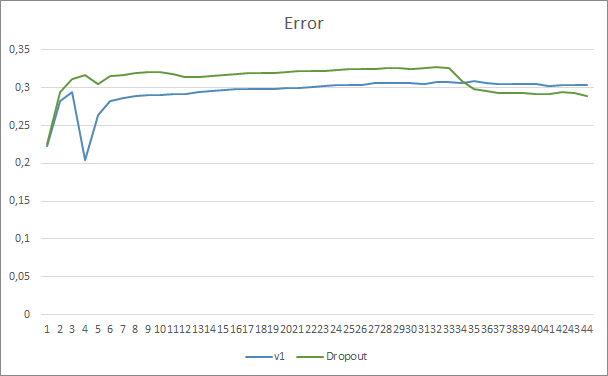The missed pattern chart also shows that the Expert Advisor utilizing the Dropout technology performs better. This chart provides even more details. The Expert Advisor using Dropout immediately shows a tendency towards a decrease in gaps. On the contrary, the Expert Advisor without dropout gradually increases missed patters areas.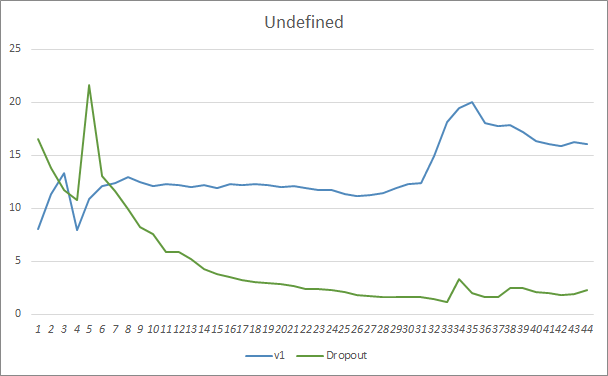Prediction hit charts of both Expert Advisors are quite close. After 44 epochs of training, the EA with Dropout is only better by 0.5%.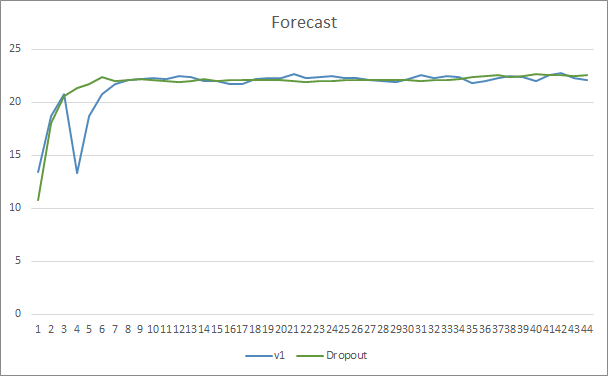### Conclusion

In this article, we started considering methods for increasing the convergence of neural networks and got acquainted with one of such methods, Dropout. The method has been added to one of our previous Expert Advisors. The efficiency of this method was shown in the EA tests. Of course, the use of this method can increase the neural network training costs. But these costs are covered by the increased efficiency of the final result.

I invite everyone to try this method and evaluate its effectiveness.

### Programs Used in the Article

# Issued to Type Description
1 Fractal_OCL_AttentionMLMH.mq5   Expert Advisor An Expert Advisor with the classification neural network (3 neurons in the output layer) using the GTP architecture, with 5 attention layers
2 Fractal_OCL_AttentionMLMH_d.mq5 Expert Advisor   An Expert Advisor with the classification neural network (3 neurons in the output layer) using the GTP architecture, with 5 attention layers + Dropout
3 NeuroNet.mqh Class library A library of classes for creating a neural network
4 NeuroNet.cl Code Base OpenCL program code library
5 NN.chm HTML Help A compiled Library Help CHM file.

Translated from Russian by MetaQuotes Software Corp.
Original article: https://www.mql5.com/ru/articles/9112

Attached files |
MQL5.zip (2459.71 KB)

#### Other articles by this author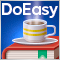Other classes in DoEasy library (part 66): MQL5.com Signals collection class
In this article, I will create the signal collection class of the MQL5.com Signals service with the functions for managing signals. Besides, I will improve the Depth of Market snapshot object class for displaying the total DOM buy and sell volumes.Prices and Signals in DoEasy library (part 65): Depth of Market collection and the class for working with MQL5.com Signals
In this article, I will create the collection class of Depths of Market of all symbols and start developing the functionality for working with the MQL5.com Signals service by creating the signal object class.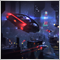Brute force approach to pattern search (Part IV): Minimal functionality
The article presents an improved brute force version, based on the goals set in the previous article. I will try to cover this topic as broadly as possible using Expert Advisors with settings obtained using this method. A new program version is attached to this article.Prices in DoEasy library (part 64): Depth of Market, classes of DOM snapshot and snapshot series objects
In this article, I will create two classes (the class of DOM snapshot object and the class of DOM snapshot series object) and test creation of the DOM data series.